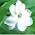## Search This Blog

THE BEAUTY OF MATHEMATICS- Collected from The Internet and Various Books to enrich The students and Teachers. SUPPORT with YOUR COMMENTS ...

## Tuesday, June 22, 2010

### SIMPLE MATH PUZZLES

PUZZLE 8

What mathematical symbol can be put between 5 and 9, to get a number bigger than 5 and smaller than 9?

PUZZLE 9

The following multiplication example uses every digit from 0 to 9 once (not counting the intermediate steps). Fill in the missing numbers.
7 _ _  *   4 _  =  _ _ _ _ _

PUZZLE 10

Here's a simple multiplication problem in which each letter represents a different digit. Can you solve it?
IF * AT = FIAT

PUZZLE 11:

Write down the next number in this series:

18,46,94,63,52, ?

PUZZLE12:

Find the next letter in the series:

O,T,T,F,F,S,S,E,?

PUZZLE 13:

How many two-digit positive whole numbers are there?

PUZZLE 14:

Find four consecutive prime numbers that add up to 220.

SOLUTIONS

PUZZLE 8 : A Decimal Point. 5.9

PUZZLE 9 : 715 * 46 = 32890

PUZZLE 10 : IF = 41. AT = 35. FIAT = 1435.

PUZZLE 11 : 61. Each is a perfect square read from back to front

PUZZLE 12 : N –  The series is the first letter of the numbers 1, 2,3,4,5,6,7,8, ? .so the next letter is N the first letter of Nine.  1[One],2[Two], 3 [Three], … 8[Eight], 9 [Nine].

PUZZLE 13: 90

PUZZLE 14:  47,53,59,61.
FOR

1.What an intersting blog Malini. Appreicate it. I found your blog through MSI. Keep up the good work.

2.3.VERY INTERESTING AND USEFUL BLOG, MALINI/MADAM,

I AM NOT A TEACHER NOR A STUDENT, BUT I HAVE A GREAT THIRST FOR PUZZLES, BRAIN TEASERS, AND FUN WITH MATHEMATICAL FIGURES , PALINDROMES, TONGUE TWISTERS ETC.
KINDLY KEEP UP THE EXCELLENT WORK, WIHCH IS VERY USEFUL FOR THE STUDENTS AND MATHS LOVERS

With Warm Wishes... Neelakantan CS, Palakkad

4.VERY INTERESTING, N I C E AND USEFUL BLOG. EXPECT MUCH MORE INTERESTING FACTS (REGARDING MATHS) AND PUZZLES.